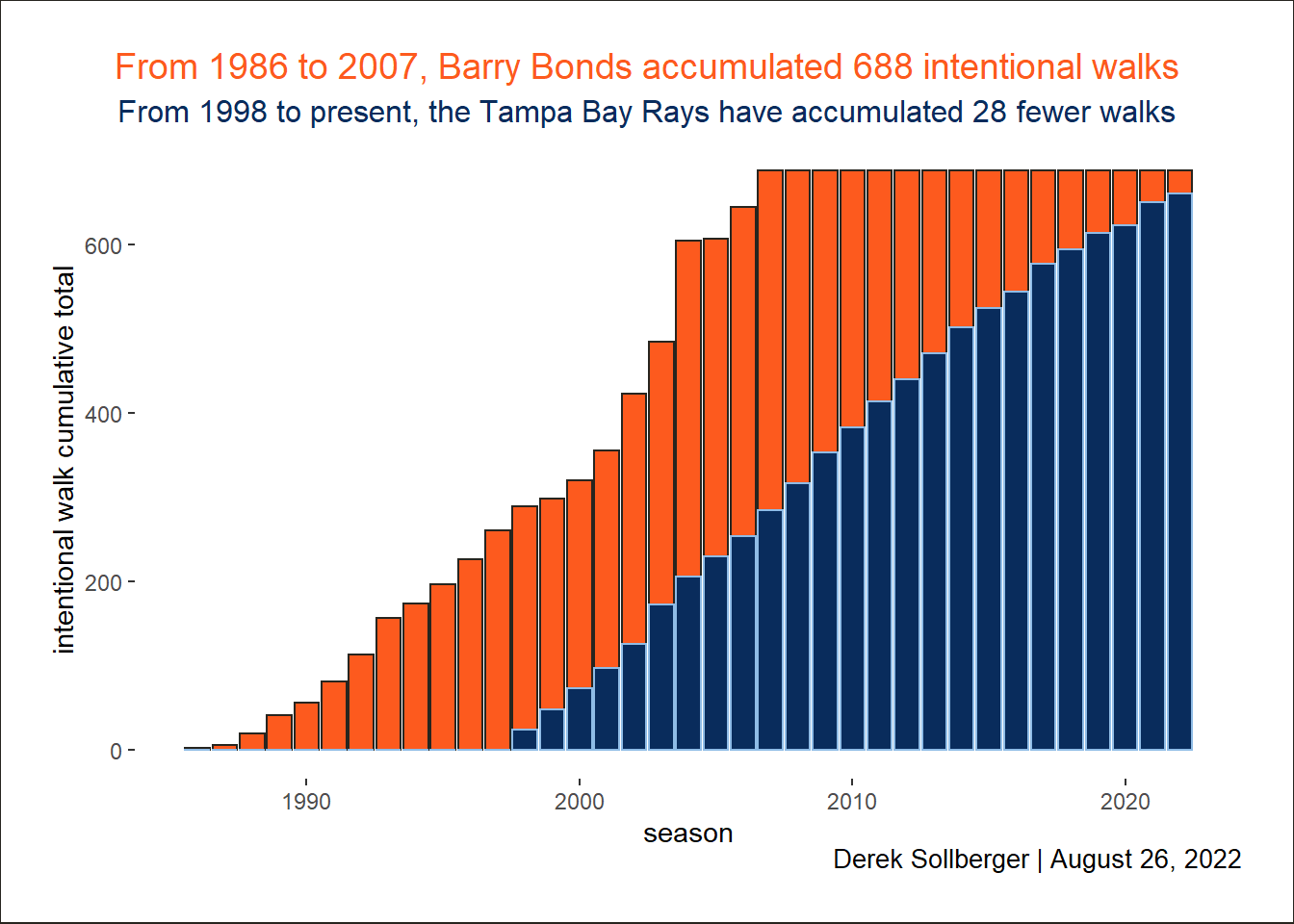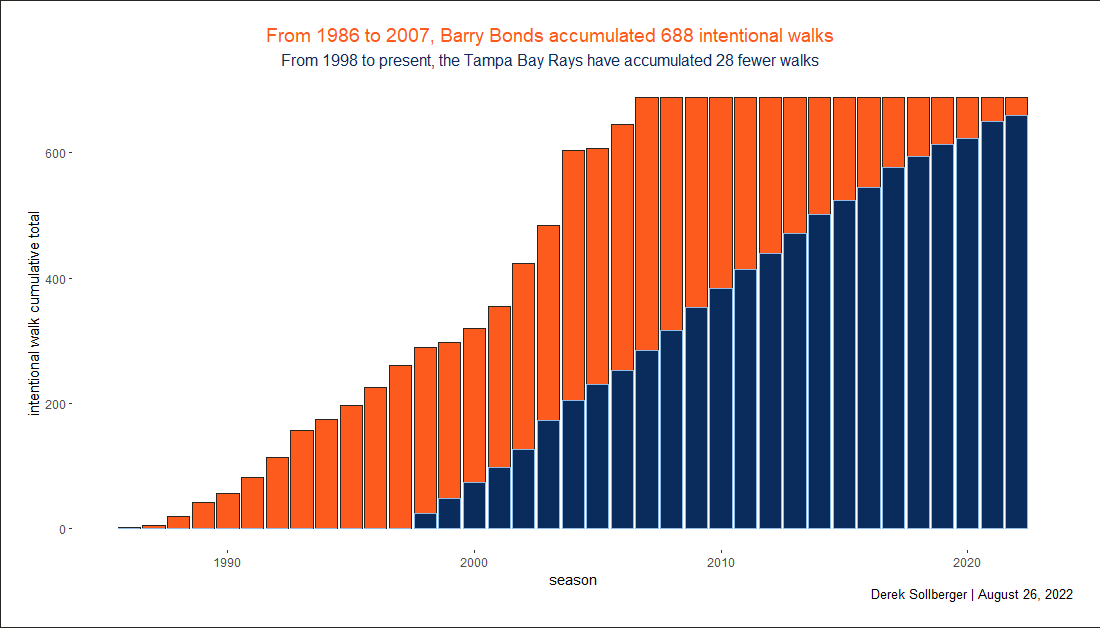# Intentional Walks

baseball
Author

Derek Sollberger

Published

Invalid Date

Today’s exploration and visualization practive was inspired by this tweet:

“Barry Bonds has over 200 more intentional walks than the Rays entire franchise.” — Cespedes Family BBQ, Dec. 2, 2013

“Almost nine years later and Bonds still has 28 more intentional walks than the Rays franchise.” — Jim Passon, Aug. 25, 2022

``````library("ggtext")
library("tidyverse")``````
``── Attaching packages ─────────────────────────────────────── tidyverse 1.3.1 ──``
``````✔ ggplot2 3.3.6     ✔ purrr   0.3.4
✔ tibble  3.1.7     ✔ dplyr   1.0.9
✔ tidyr   1.2.0     ✔ stringr 1.4.0
✔ readr   2.1.2     ✔ forcats 0.5.1``````
``````── Conflicts ────────────────────────────────────────── tidyverse_conflicts() ──

## Gathering the Data

At first, I thought that I could reasonably type in all of the data, but Bonds’ career was quite long. I copy-and-pasted the batting table from Baseball Reference and focused on the `Year` and `IBB` columns.

The franchise page for the Tampa Bay Rays did not have intentional walks quickly accessible, so I simply went through the year-by-year pages and gathered the `IBB` total (fortunately easy to find visually as the last column).

``````df_bonds <- readxl::read_xlsx("ibb.xlsx", sheet = "Bonds")

## Data Wrangling

While I could probably affect the horizontal axis later in a `ggplot` visualization, I prefer today to have the data in one data frame. Let me merge the data.

``````df <- df_bonds |>
full_join(df_rays, by = "Year") |>
rename("IBB_Bonds" = "IBB.x",
"IBB_Rays" = "IBB.y")``````

The naturally missing values probably would not affect the calculations much, but for peace of mind, let us impute those values to be zeroes.

``````df\$IBB_Bonds[is.na(df\$IBB_Bonds)] <- 0
df\$IBB_Rays[is.na(df\$IBB_Rays)]   <- 0``````

In the spirit of the tweet, we will need to talk about cumulative totals.

``````df <- df |>
mutate(IBB_Bonds_total = cumsum(IBB_Bonds),
IBB_Rays_total  = cumsum(IBB_Rays))``````

## Data Visualization

While line graphs (with areas filled) would probably be the most visually appealing, it might be better to treat seasons as discrete entries in a bar plot.

``````df |>
ggplot() +
geom_bar(aes(x = Year, y = IBB_Bonds_total),
stat = "identity")``````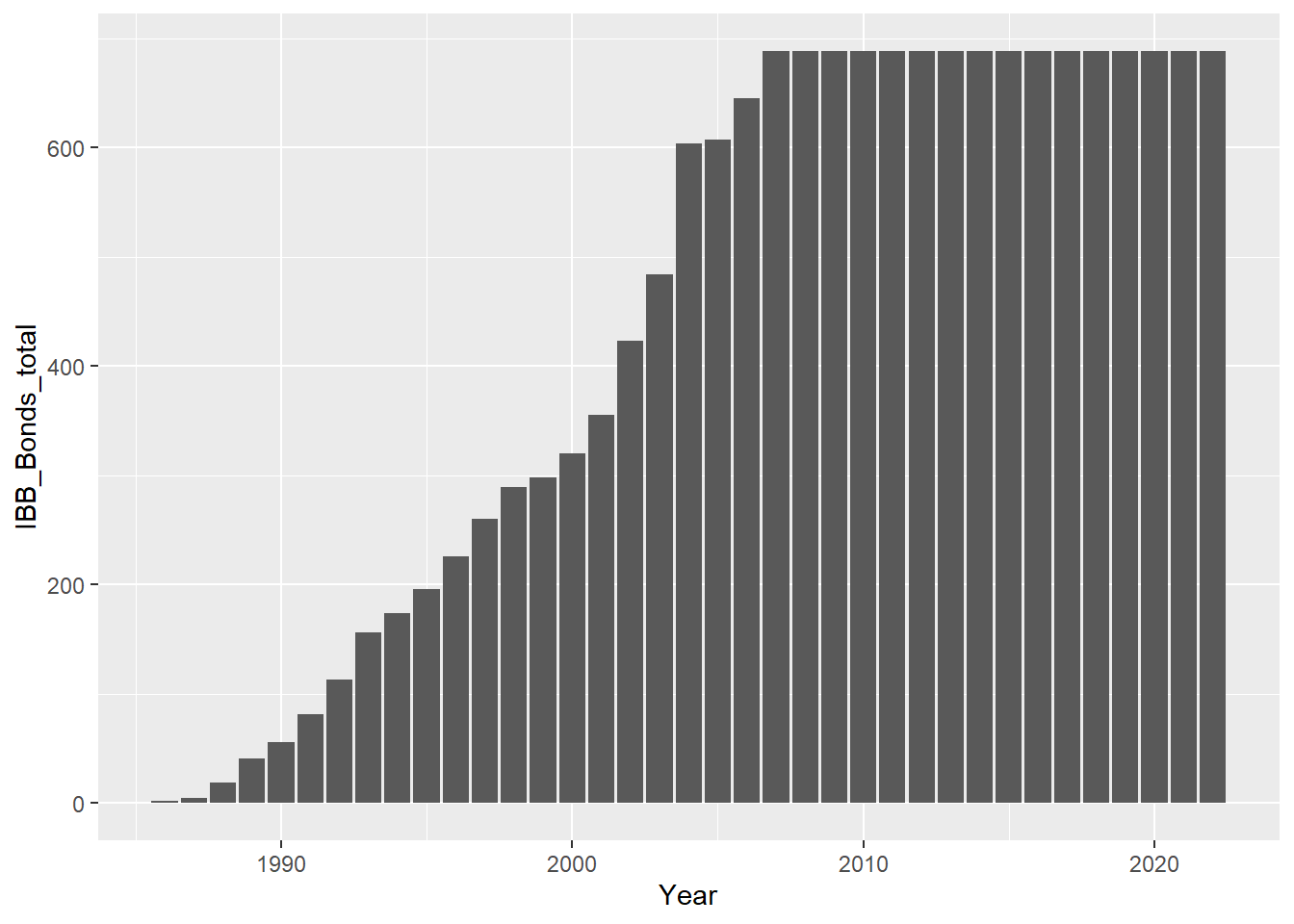Now I am curious what the bars will look like in team colors.

``````baseplot <- df |>
ggplot() +

# https://teamcolorcodes.com/san-francisco-giants-color-codes/
geom_bar(aes(x = Year, y = IBB_Bonds_total),
color = "#27251F", fill = "#FD5A1E",
stat = "identity") +

# https://teamcolorcodes.com/tampa-bay-rays-color-codes/
geom_bar(aes(x = Year, y = IBB_Rays_total),
color = "#8FBCE6", fill = "#092C5C",
stat = "identity")

# print
baseplot``````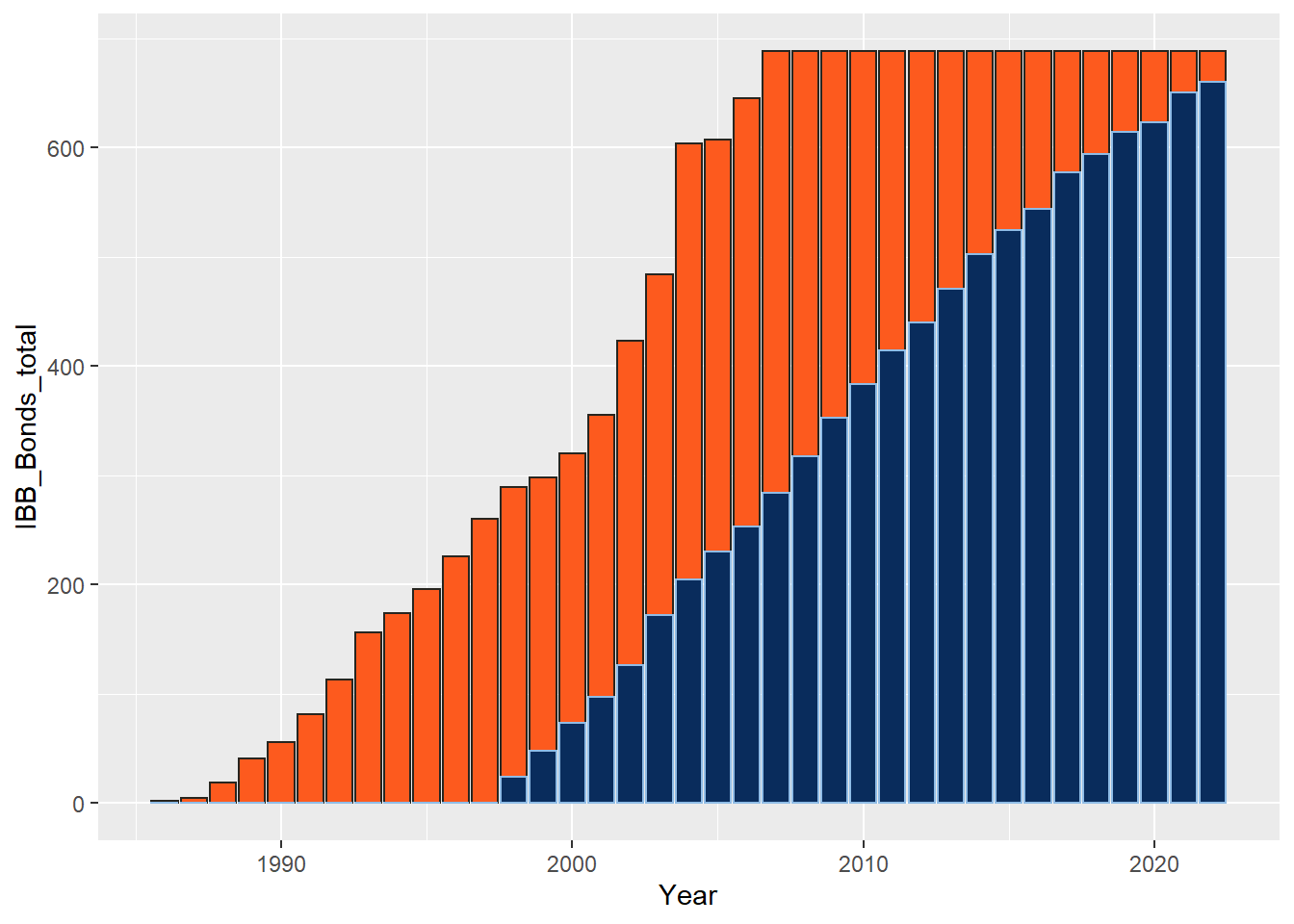Moving toward aesthetic beauty, I will update some of the theme elements.

``````# some ideas from
# https://github.com/nikopech/TidyTuesday/blob/master/R/2022-08-09/2022_08_09_ferris_wheels.R

current_plot <- baseplot +
labs(title = "",
subtitle = "",
caption = "Derek Sollberger | August 26, 2022",
x = "season",
y = "intentional walk cumulative total") +
theme(legend.position = "none",
panel.background = element_blank(),
plot.background = element_rect(
fill = "#FFFFFF",
color = "#27251F"
),
plot.title = element_markdown(face = "italic",
margin = margin(b = 5),
size = 14),
plot.title.position = "plot",
plot.subtitle = element_markdown(face = "italic",
margin = margin(b = 5),
size = 12),
plot.caption = element_markdown(margin = margin(t = 0),
size = 10),
plot.caption.position = "plot",
plot.margin = margin(50, 50, 50, 50))

# print
current_plot``````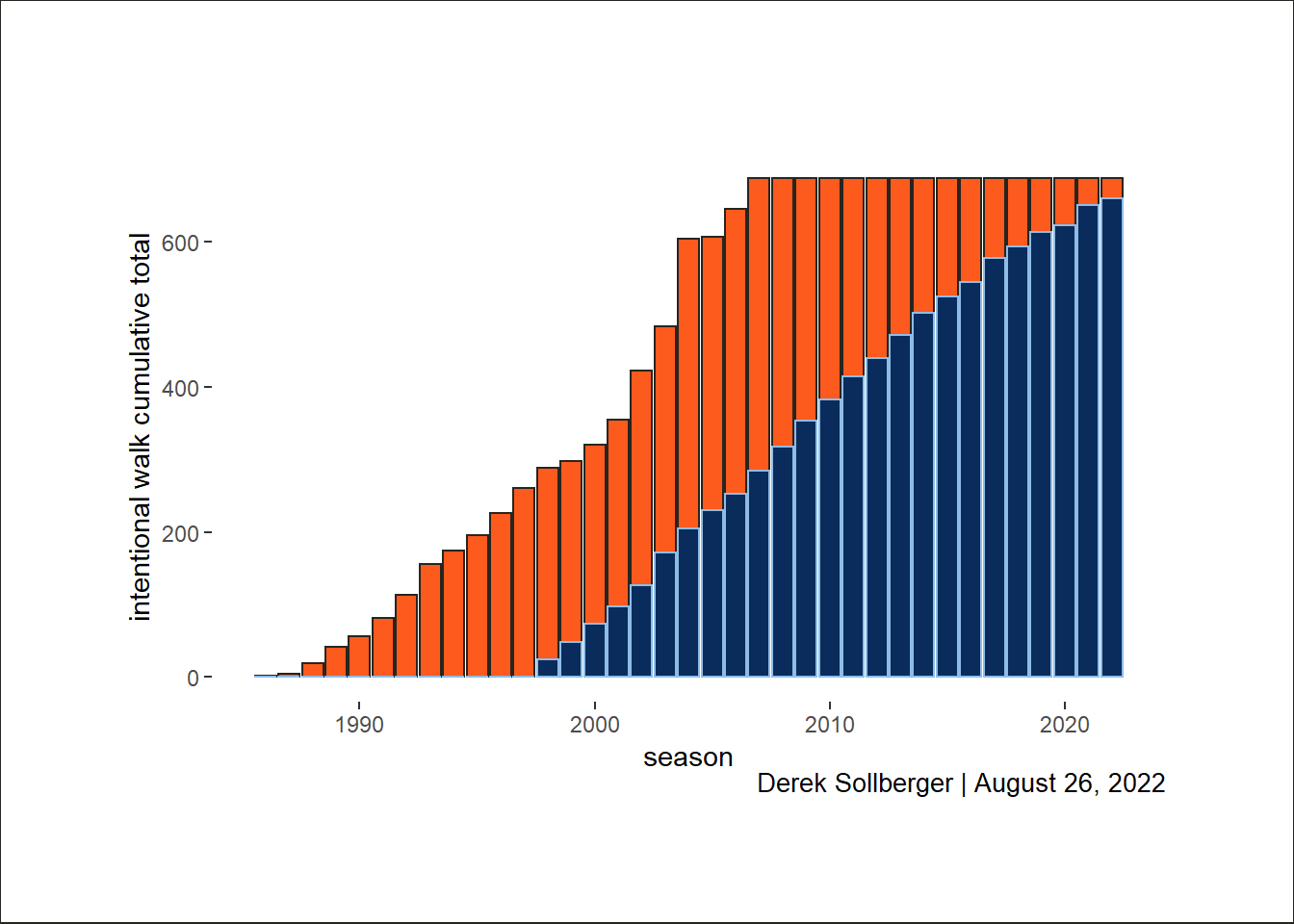Now, I am going to attempt to add arrows (and later: labels) to highlight certain areas of the graph. This is still very new to me, so fingers crossed.

``````current_plot +
# In 2004, Barry Bonds received 120 intentional walks
annotate(color = "#FD5A1E",
geom = "curve",
size = 0.5,
x = 1999, xend = 2004,
y = 800, yend = 604) +
geom_textbox(aes(x = 1999, y = 800,
color = "#000000",
label = "In 2004, Barry Bonds received 120 intentional walks"),
size = 2) +

# Start of Barry Bonds' MLB career
annotate(color = "#FD5A1E",
geom = "curve",
size = 0.5,
x = 1988, xend = 1986,
y = 200, yend = 0) +
geom_textbox(aes(x = 1988, y = 200,
color = "#000000",
label = "Start of Barry Bonds' MLB career"),
size = 2)``````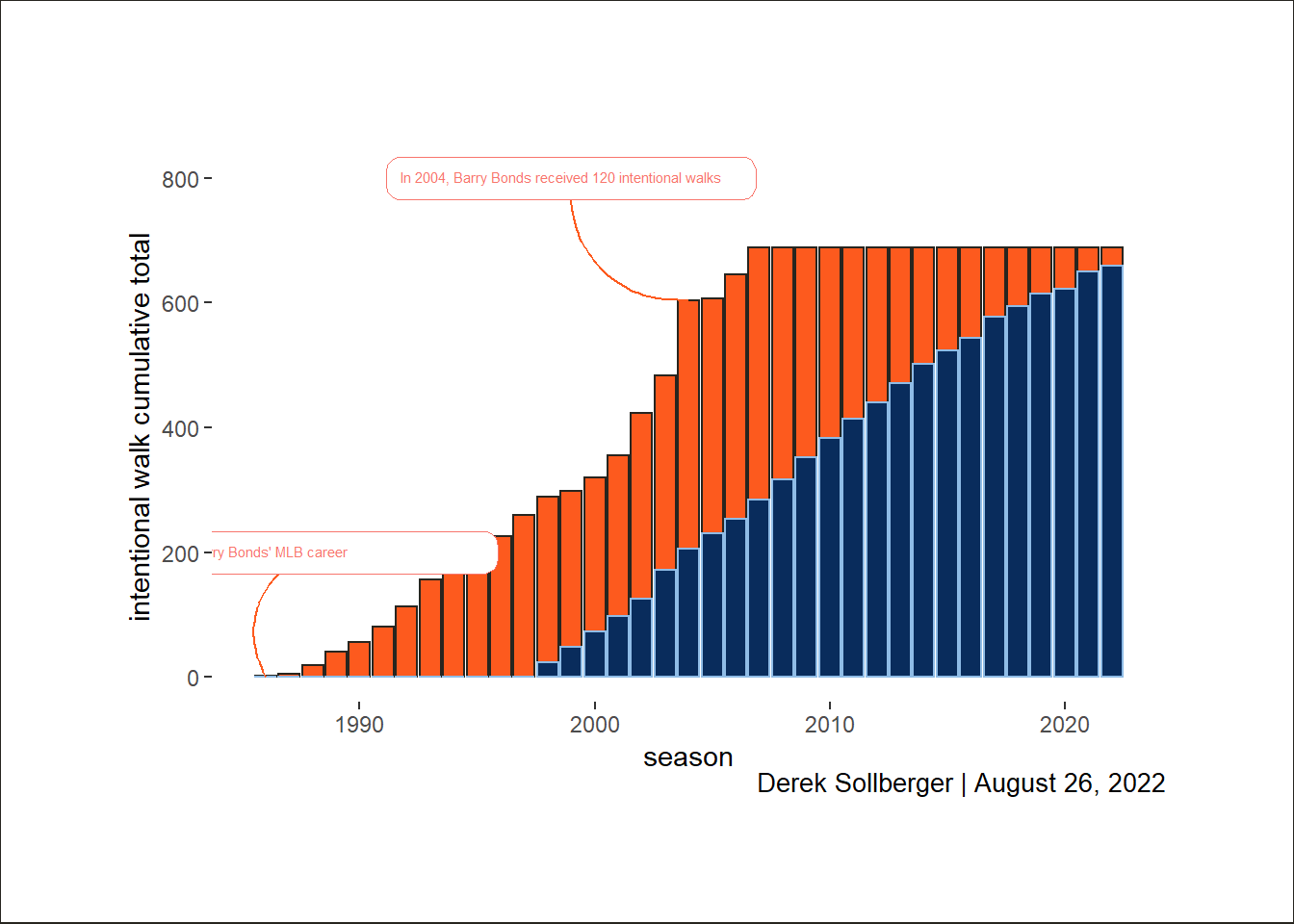``````# print
# current_plot``````

At the moment, I am having difficulty getting labels to naturally appear beyond the `panel`.

Instead, I will focus on the title and caption.

``````# some ideas from
# https://github.com/nikopech/TidyTuesday/blob/master/R/2022-08-09/2022_08_09_ferris_wheels.R

current_plot <- baseplot +
labs(title = "From 1986 to 2007, Barry Bonds accumulated 688 intentional walks",
subtitle = "From 1998 to present, the Tampa Bay Rays have accumulated 28 fewer walks",
caption = "Derek Sollberger | August 26, 2022",
x = "season",
y = "intentional walk cumulative total") +
theme(legend.position = "none",
panel.background = element_blank(),
plot.background = element_rect(
fill = "#FFFFFF",
color = "#27251F"
),
plot.title = element_text(color = "#FD5A1E", size = 14, hjust = 0.5),
plot.title.position = "plot",
plot.subtitle = element_text(color = "#092C5C", size = 12, hjust = 0.5),
plot.caption = element_markdown(margin = margin(t = 0),
size = 10),
plot.caption.position = "plot",
plot.margin = margin(20, 20, 20, 20))

# print
current_plot``````